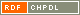Naslov: On 2-fold covers of graphs Feng, Yan-Quan (Avtor)Kutnar, Klavdija (Avtor)Malnič, Aleksander (Avtor)Marušič, Dragan (Avtor)http://dx.doi.org/10.1016/j.jctb.2007.07.001 Angleški jezik Delo ni kategorizirano 1.01 - Izvirni znanstveni članek IAM - Inštitut Andrej Marušič A regular covering projection ▫$\wp : \widetilde{X} \to X$▫ of connected graphs is ▫$G$▫-admissible if ▫$G$▫ lifts along ▫$\wp$▫. Denote by ▫$\tilde{G}$▫ the lifted group, and let CT▫$(\wp)$▫ be the group of covering transformations. The projection is called ▫$G$▫-split whenever the extension ▫{$\mathrm{CT}}(\wp) \to \tilde{G} \to G$▫ splits. In this paper, split 2-covers are considered, with a particular emphasis given to cubic symmetric graphs. Supposing that ▫$G$▫ is transitive on ▫$X$▫, a ▫$G$▫-split cover is said to be ▫$G$▫-split-transitive if all complements ▫$\tilde{G} \cong G$▫ of CT▫$(\wp)$▫ within ▫$\tilde{G}$▫ are transitive on ▫$\widetilde{X}$▫; it is said to be ▫$G$▫-split-sectional whenever for each complement ▫$\tilde{G}$▫ there exists a ▫$\tilde{G}$▫-invariant section of ▫$\wp$▫; and it is called ▫$G$▫-split-mixed otherwise. It is shown, when ▫$G$▫ is an arc-transitive group, split-sectional and split-mixed 2-covers lead to canonical double covers. Split-transitive covers, however, are considerably more difficult to analyze. For cubic symmetric graphs split 2-cover are necessarily canonical double covers (that is, no ▫$G$▫-split-transitive 2-covers exist) when ▫$G$▫ is 1-regular or 4-regular. In all other cases, that is, if ▫$G$▫ is ▫$s$▫-regular, ▫$s=2,3$▫ or ▫$5$▫, a necessary and sufficient condition for the existence of a transitive complement ▫$\tilde{G}$▫ is given, and moreover, an infinite family of split-transitive 2-covers based on the alternating groups of the form ▫$A_{12k+10}$▫ is constructed. Finally, chains of consecutive 2-covers, along which an arc-transitive group ▫$G$▫ has successive lifts, are also considered. It is proved that in such a chain, at most two projections can be split. Further, it is shown that, in the context of cubic symmetric graphs, if exactly two of them are split, then one is split-transitive and the other one is either split-sectional or split-mixed. graph theory, graphs, cubic graphs, symmetric graphs, ▫$s$▫-regular group, regular covering projection 2008 str. 324-341 Vol. 98, no. 2 0095-8956 519.17 25248871193 13Gradivo ni uvrščeno v področja.

Skupna ocena: (0 glasov) Ocenjevanje je dovoljeno samo prijavljenim uporabnikom.Postavite miškin kazalec na naslov za izpis povzetka. Klik na naslov izpiše podrobnosti ali sproži prenos.

## Sekundarni jezik

Jezik: Angleški jezik teorija grafov, grafi, kubični grafi, simetrični grafi, ▫$s$▫-regularna grupa, regularna krovna projekcija

## Komentarji

Dodaj komentar

izpis_zaKomentiranje

Komentarji (0)
 0 - 0 / 0 Ni komentarjev!

Nazaj